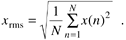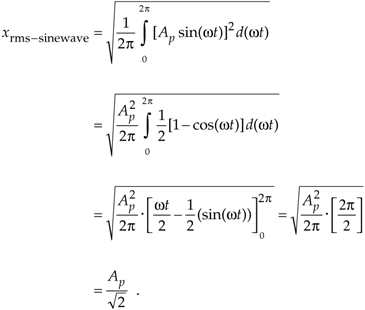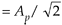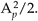# Section D.2. STANDARD DEVIATION, OR RMS, OF A CONTINUOUS SINEWAVE

### D 2 STANDARD DEVIATION, OR RMS, OF A CONTINUOUS SINEWAVE

For sinewaves, electrical engineers have taken the square root of Eq. (D-3), with xave = 0, and defined a useful parameter, called the rms value, that's equal to the standard deviation. For discrete samples, that parameter, xrms, is defined asThe xrms in Eq. (D-6), obtained by setting xave = 0 in Eq. (D-5), is the square root of the mean (average) of the squares of the sequence x(n). For a continuous sinusoid x(t) = Apsin(2pft) = Apsin(wt) whose average value is zero, xrms is xrms-sinewave defined as

Equation D-7This xrms-sinewaveexpression is a lot easier to use for calculating average power dissipation in circuit elements than taking the integral of more complicated expressions for instantaneous power dissipation. The variance of a sinewave is, of course, the square of Eq. (D-7), orWe've provided the equations for the mean (average) and variance of a sequence of discrete values, introduced an expression for the standard deviation or rms of a sequence, and given an expression for the rms of a continuous sinewave. The next question is "How can we characterize random functions for which there are no equations to predict their values and we have no discrete sample values with which to work?" The answer is that we must use probability density functions.

URL http://proquest.safaribooksonline.com/0131089897/app04lev1sec2AmazonPrev don't be afraid of buying books Next